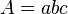# Ultraviolet–visible absorption facts for kids

Kids Encyclopedia Facts

UV-visible absorption is a process where a molecule absorbs ultraviolet or visible light that excites electrons (makes them high energy). This energy causes an electronic transition from a ground state (non excited) to an excited state.

This is used in a technique of absorption spectroscopy known as UV-visible spectroscopy.

## Energy absorption

Different molecules will absorb different wavelengths of light. The wavelengths of light for UV-visible absorption are from about 200 nanometers to 800 nanometers. This is a section of the electromagnetic spectrum pictured.

Absorption of light starts with energy of a certain wavelength in this UV-visible region being exposed to a molecule. The light/energy then excites the ground state (non excited) outer or valence electrons to an excited state (high energy). The outcome of this can be measured by a UV-visible spectrophotometer. The data is shown as a spectrum with absorption versus wavelength. This pattern can be used to learn properties of the molecule. Only certain molecules can absorb light in this region.

## Chromophores

Molecules that absorb light at these wavelengths are called chromophores. Chromophores are functional groups of a molecule that absorb light in this UV-Visible region. They are most of the time characterized by delocalized pi electrons. Pi electrons refer to a type of bond that occurs between electron orbitals called pi orbitals. When many of these pi bonds exist in a molecule this allows electrons to be delocalized or spread out across a molecule. An example of this type of molecule is pictured below. Many dyes (colored molecules) are characterized by these delocalized pi electrons and their color. These molecules can be used for pH indicators to determine if a solution is acidic or basic. The addition of acid or base disrupts the delocalized pi electrons. This disruption causes a color change.

## Beer’s Law

The absorption of a molecule can be used to determine the concentration of the molecule in solution. To find concentration, Beer’s law is used. This is a mathematical relationship shown by the equation below.$A=abc$
A= absorption, a=absorptivity coefficient, b=pathlength, c=concentration

The components of Beer’s Law can be determined by tests. They can also be referenced in literature. If the absorption is measured by a UV-visible spectrophotometer (instrument to measure absorption and wavelength of molecules), pathlength (b) is related to the sample container used in the test. The sample container (named a cuvette) is made of a material that does not absorb light in this region. The thickness of this cuvette is a known value. This is the pathlength the light will travel through to reach the sample. The molar absorptivity coefficient can be calculated by measuring the absorption of a sample of a known concentration and known pathlength. The absorptivity coefficient units are dependent on the pathlength and concentration units. Molar absorptivity units are L mol-1 cm-1 when the concentration units are molarity. The absorptivity is a measure of how strongly a molecule absorbs light at a particular wavelength. Once this constant is determined, unknown concentrations can be determined from this relationship.

## Images for kidsUltraviolet–visible absorption Facts for Kids. Kiddle Encyclopedia.